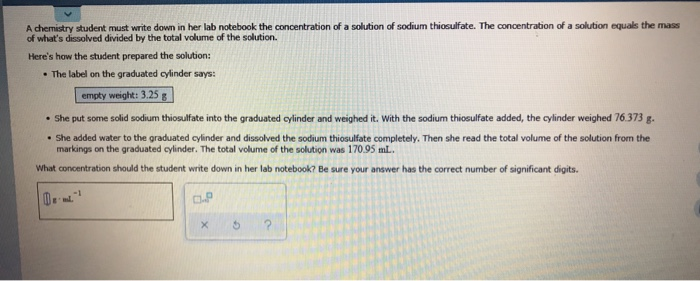# A chemistry student must write down in her lab notebook the concentration of a solution of...

###### Question:A chemistry student must write down in her lab notebook the concentration of a solution of sodium thiosulfate. The concentration ofa solution equals the mass of what's dissolved divided by the total volume of the solution. Here's how the student prepared the solution: The label on the graduated cylinder says: empty weight: 3.25g She put some solid sodium thiosulfate into the graduated cylinder and weighed it. With the sodium thiosulfate added, the cylinder weighed 76.373 g. She added water to the graduated cylinder and dissolved the sodium thiosulfate completely. Then she read the total volume of the solution from the markings on the graduated cylinder. The total volume of the solution was 170.95 mL What concentration should the student write down in her lab notebook? Be sure your answer has the correct number of significant digits. -1 CP

#### Similar Solved Questions

##### How do you simplify (s^-3 t^-5)/(s^2 t^3)^-1?
How do you simplify (s^-3 t^-5)/(s^2 t^3)^-1?...
##### Please answer question 3 ABC Enterprises began business on October 1, 2019. Below is the monthly...
please answer question 3 ABC Enterprises began business on October 1, 2019. Below is the monthly payroll for ABC Enterprises, Inc. All employees are on salary and receive the same amount each month. Last Name First Gross Fed Inc Medical Medical Insurance 401K Earnings $12,000.00$ 2,280.00 \$600.00...
##### How do you find h(-1) given h(x)=-x^2-3?
How do you find h(-1) given h(x)=-x^2-3?...